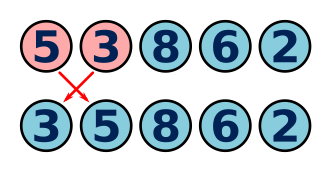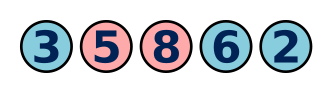# Bubble sort algorithm

Martin McBride, 2017-01-09
Tags sort bubble sort
Categories algorithms sort

Bubble sort is another simple sorting algorithm.

## Bubble sort first pass

Here is the simplest version of a bubble sort. We are going to sort these cards in ascending order:The process is quite simple. Starting from the left, we take each pair of numbers. If the pair of numbers is in the wrong order, we swap them. First we take the pair 5 and 3 - they are in the wrong order (the 5 should be on the right because it is higher) so we swap them:Now we take the next pair, 5 and 8 They are in the correct order, so leave them alone:Then we take the pair 8 and 6 - they are in the wrong order, because 8 is greater than 6, so we swap them:Finally we take the pair 8 and 2 - again, they are in the wrong order, so we swap them:## Completing the algorithm

Here is what the list looks like after the first pass. Notice that the numbers are not yet in the correct order, but the final number, 8, is in the correct place:

3, 5, 6, 2, 8


Now we repeat the same process again - starting at the left, we compare each pair of numbers, and swap them if necessary. Here is what the new list looks like. Notice that the numbers are still not in the correct order, but the final two numbers, 8 and 6, are in the correct places:

3, 5, 2, 6, 8


Following this pattern, if we run the process 4 times, the last 4 numbers will be in the correct places:

2, 3, 5, 6, 8


If you think about it, if the last 4 numbers are in the correct places, the first number must also be in the correct place, because that is the only place left. So to fully sort a list of 5 values, we must repeat the sorting pass 4 times.

In general, to fully sort a list of n values, we must repeat the sorting pass (n-1) times. Here is the pseudo code for the algorithm:

FOR i = 0 TO LENGTH(k)-2
FOR j = 0 TO LENGTH(k)-2
IF k[j]>k[j+1]:
SWAP(k[j], k]j+1])


## An optimisation

There is something you can do to make the algorithm a little bit faster. You will notice that after the first path, the list is:

3, 5, 6, 2, 8


When we apply the second pass of the algorithm, we don't need to process all 5 elements because the final element is already sorted. We can just process the first 4 elements. On the third pass, the list looks like this:

3, 5, 2, 6, 8


This time we only need to process the first 3 elements. Overall, if we avoid processing the extra elements, the algorithm can be almost twice as fast.

The code change is very simple. On the i'th pass through the loop, we don't need to process the final i elements, so the j loop becomes:

    FOR j = 0 TO LENGTH(k)-2-i


Making the final code:

FOR i = 0 TO LENGTH(k)-2
FOR j = 0 TO LENGTH(k)-2-i
IF k[j]>k[j+1]:
SWAP(k[j], k]j+1])